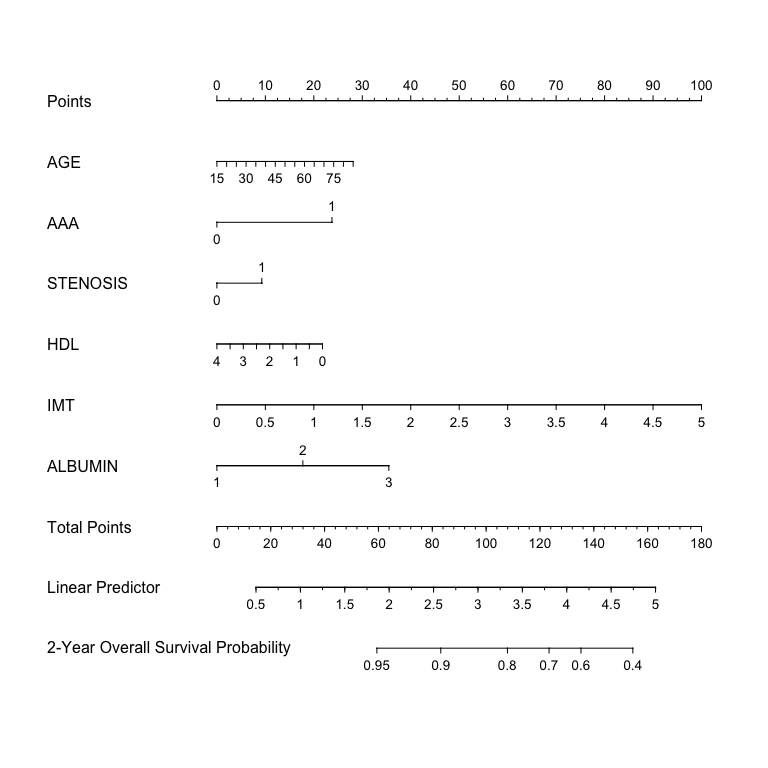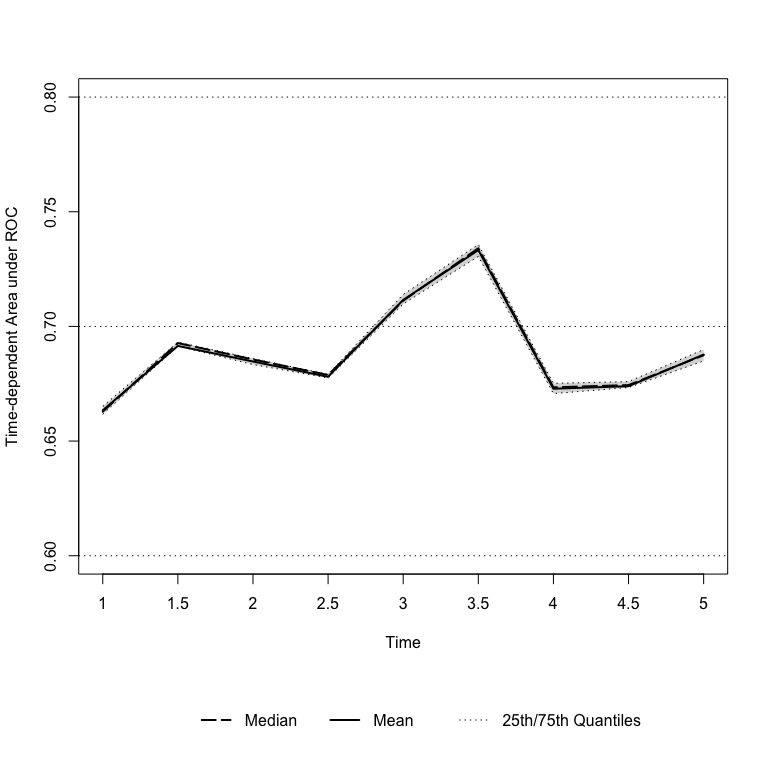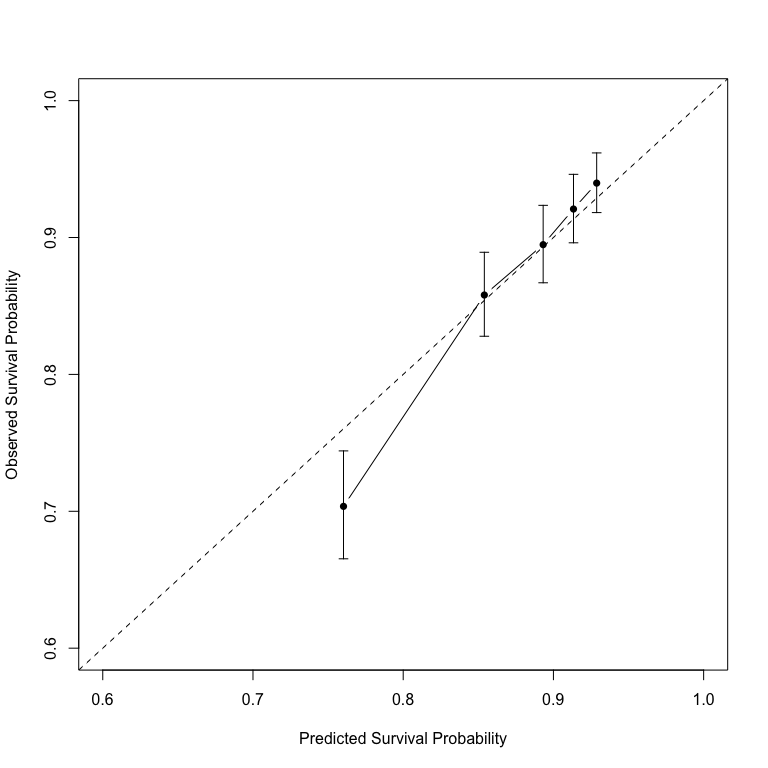# 1 Introduction

It is a challenging task to model the emerging high-dimensional clinical data with survival outcomes. For its simplicity and efficiency, penalized Cox models is significantly useful for accomplishing such tasks.

hdnom streamlines the workflow of high-dimensional Cox model building, model validation, model calibration, and nomogram plotting. To load the package in R, simply type:

library("hdnom")

# 2 Build Penalized Cox Models

Penalized Cox models usually needs parameters tuning. For example, the elastic-net model requires to tune the $$\ell_1$$-$$\ell_2$$ penalty trade-off parameter $$\alpha$$, and the regularization parameter $$\lambda$$.

To free the users from the tedious and error-prone parameter tuning process, hdnom provides several functions for automatic parameter tuning and model selection, including the following model types:

• hdcox.lasso() - lasso model;
• hdcox.alasso() - adaptive lasso model;
• hdcox.enet() - elastic-net model;
• hdcox.aenet() - adaptive elastic-net model.

In the next, we will use the imputed SMART study data (Steyerberg 2008) to demonstrate a complete process of model building, nomogram plotting, model validation, and model calibration with hdnom.

Load the smart dataset:

data("smart")
x = as.matrix(smart[, -c(1, 2)])
time = smart$TEVENT event = smart$EVENT

library("survival")
y = Surv(time, event)

The dataset contains 3873 observations with corresponding survival outcome (time, event). 27 clinical variables (x) are available as the predictors. See ?smart for detailed explanation of the variables.

Fit a penalized Cox model by adaptive elastic-net regularization, with hdcox.aenet:

# Enable parallel parameter tuning
suppressMessages(library("doParallel"))
registerDoParallel(detectCores())

aenetfit = hdcox.aenet(x, y, nfolds = 10, rule = "lambda.1se",
seeds = c(5, 7), parallel = TRUE)
names(aenetfit)
##  "enet_best_alpha"   "enet_best_lambda"  "enet_model"
##  "aenet_best_alpha"  "aenet_best_lambda" "aenet_model"
##  "pen_factor"

The adaptive elastic-net model includes two estimation steps. The selected best $$\alpha$$, the selected best $$\lambda$$, the model fitted for each estimation step, and the penalty factor for the model coefficients in the second estimation step are all stored in the aenetfit list object.

For building nomograms, it is also possible to employ the cv.glmnet() and glmnet() functions in the glmnet package (Simon et al. 2011) directly to tune and fit penalized Cox models, as long as the final model object is returned by glmnet().

# 3 Plot Nomograms

Before plotting the nomogram, we need to extract some necessary information about the model, namely, the model object and model parameters, from the result of the last step:

fit    = aenetfit$aenet_model alpha = aenetfit$aenet_best_alpha
lambda = aenetfit$aenet_best_lambda adapen = aenetfit$pen_factor

To plot the nomogram, first we make x available as a datadist object for the rms package (Harrell 2013), then generate a hdnom.nomogram object with hdnom.nomogram(), and finally plot the nomogram:

suppressMessages(library("rms"))
x.df = as.data.frame(x)

nom = hdnom.nomogram(fit, x, time, event, x.df,
lambda = lambda, pred.at = 365 * 2,
funlabel = "2-Year Overall Survival Probability")
plot(nom)According to the nomogram, the adaptive elastic-net model selected 6 variables from the original set of 27 variables, effectively reduced the model complexity.

As the internal information of the nomogram, the point-linear predictor unit mapping, and total points-survival probability mapping, can be viewed by directly printing the nom object.

# 4 Model Validation

It is a common practice to utilize resampling methods to validate the predictive performance of a Cox model. Bootstrap, k-fold cross-validation, and repeated k-fold cross-validation are the mostly employed resampling methods for such purpose. hdnom.validate allows us to assess the model performance by time-dependent AUC (Area Under the ROC Curve) with the above three resampling methods.

Here, we validate the adaptive elastic-net model performance by time-dependent AUC with bootstrap resampling, at every half year from the first year to the fifth year:

set.seed(11)
val = hdnom.validate(x, time, event,
alpha = alpha, lambda = lambda, pen.factor = adapen,
method = "bootstrap", boot.times = 10,
tauc.type = "UNO", tauc.time = seq(1, 5, 0.5) * 365,
trace = FALSE)
val
## High-Dimensional Cox Model Validation Object
## Validation method: bootstrap
## Bootstrap samples: 10
## glmnet model alpha: 0.15
## glmnet model lambda: 2.559313e+12
## glmnet model penalty factor: specified
## Time-dependent AUC type: UNO
## Evaluation time points for tAUC: 365 547.5 730 912.5 1095 1277.5 1460 1642.5 1825
summary(val)
## Time-Dependent AUC Summary at Evaluation Time Points
##                365     547.5       730     912.5      1095    1277.5
## Mean     0.6634202 0.6914840 0.6847023 0.6780378 0.7115520 0.7332658
## Min      0.6589659 0.6847332 0.6799259 0.6734126 0.7085565 0.7278341
## 0.25 Qt. 0.6617228 0.6914073 0.6833705 0.6775351 0.7097986 0.7305095
## Median   0.6628115 0.6927359 0.6855877 0.6788320 0.7112025 0.7340682
## 0.75 Qt. 0.6651182 0.6931459 0.6859754 0.6790672 0.7138966 0.7357173
## Max      0.6681952 0.6948522 0.6887332 0.6806368 0.7149059 0.7376617
##               1460    1642.5      1825
## Mean     0.6728266 0.6739268 0.6877137
## Min      0.6648067 0.6675631 0.6830705
## 0.25 Qt. 0.6707031 0.6733444 0.6849454
## Median   0.6734421 0.6744008 0.6874298
## 0.75 Qt. 0.6751822 0.6759282 0.6899151
## Max      0.6779148 0.6763337 0.6936300

The mean, median, 25%, and 75% quantiles of time-dependent AUC at each time point across all bootstrap predictions are listed above. The median and the mean can be considered as the bias-corrected estimation of the model performance.

It is also possible to plot the model validation result:

plot(val, ylim = c(0.6, 0.8), xaxt.label = seq(1, 5, 0.5))It seems that the bootstrap-based validation result is stable: the median and the mean value at each evaluation time point are close; the 25% and 75% quantiles are also close to the median at each time point.

Cross-validation and repeated cross-validation will usually yield results with different patterns. Check ?hdnom.validate for more examples about model validation.

# 5 Model Calibration

Measuring how far the model predictions are from actual survival outcomes is known as calibration. Calibration can be assessed by plotting the predicted probabilities from the model versus actual survival probabilities.

hdnom.calibrate() provides non-resampling and resampling methods for model calibration, including direct fitting, bootstrap resampling, k-fold cross-validation and repeated cross-validation.

For example, to calibrate the model with the bootstrap method:

cal = hdnom.calibrate(x, time, event,
alpha = alpha, lambda = lambda, pen.factor = adapen,
method = "bootstrap", boot.times = 10,
pred.at = 365 * 5, ngroup = 5,
trace = FALSE)
cal
## High-Dimensional Cox Model Calibration Object
## Calibration method: bootstrap
## Bootstrap samples: 10
## glmnet model alpha: 0.15
## glmnet model lambda: 2.559313e+12
## glmnet model penalty factor: specified
## Calibration time point: 1825
## Number of groups formed for calibration: 5
summary(cal)
##   Calibration Summary Table
##   Predicted  Observed Lower 95% Upper 95%
## 1 0.7602636 0.7036008 0.6652904 0.7441173
## 2 0.8540101 0.8579952 0.8278691 0.8892176
## 3 0.8931653 0.8947697 0.8669304 0.9235030
## 4 0.9133613 0.9208065 0.8961349 0.9461573
## 5 0.9287966 0.9397485 0.9182076 0.9617947

The calibration result (median of the predicted survival probability; median of the observed survival probability estimated by Kaplan-Meier method, with 95% CI) are summarized above.

Plot the calibration result:

plot(cal, xlim = c(0.6, 1), ylim = c(0.6, 1))See ?hdnom.calibrate for more examples about model calibration.

For further information about hdnom, consult the project homepage: nomogr.am.